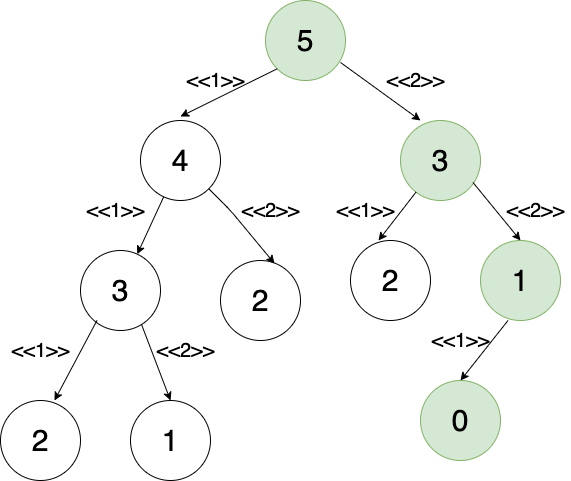1598321160

# Algorithm Problem Solving — Recursion Basics

We are going to talk about how to intuitively think about recursion.
Problem Statement
You are climbing a staircase. It takes n steps to reach to the top. Each time you can either climb 1 or 2 step(s). In how many distinct ways you can climb to the top?
This problem is a typical model for recursion. To easily visualize how you can solve this problem assume that you are on the top position already, A friend of yours is going to climb up the stairs.

#algorithms #recursion #data-structures #java #programming

## Buddha Community1605176864

## How to do Problem Solving as a Developer

In this video, I will be talking about problem-solving as a developer.

#problem solving skills #problem solving how to #problem solving strategies #problem solving #developer1625673900

## Recursion in 3 steps | Recursion Algorithms Basics - Part 1 | DSA-One Course #8

Hey guys in this video, we’ll talk about basics of Recursion. We’ll solve some basic Recursion problems using 3 Simple steps.

1. Find the Base case
2. Find the relation between problem and subproblems
3. Generalise the relation.

0:00 Overview of Recursion
2:21 Print Hello n times using Recursion
6:26 Find the sum of n Natural numbers using Recursion
9:48 Calculate pow(a,b) using Recursion

Instagram: https://www.instagram.com/Anuj.Kumar.Sharma
Telegram: https://t.me/coding_enthusiasts

Join the Telegram Group for DSA-One course: https://t.me/dsa_one

Ignore these tags:
recursion,recursion in c,recursion java,recursion explained,what is recursion,recursion examples,recursion in java,recursion program in c,recursion tree,java recursion,recursion in depth,recursion example,how recursion works,recursion algorithm,factorial recursion,recursion javascript,recursion programming,recursion explanation,recursion easy,tail recursion,head recursion,recurstion,leran recursion,recursion guide,trace recursion,learn recursion,recursion video,recursion in c++

#recursion #algorithms basics #algorithms1598321160

## Algorithm Problem Solving — Recursion Basics

We are going to talk about how to intuitively think about recursion.
Problem Statement
You are climbing a staircase. It takes n steps to reach to the top. Each time you can either climb 1 or 2 step(s). In how many distinct ways you can climb to the top?
This problem is a typical model for recursion. To easily visualize how you can solve this problem assume that you are on the top position already, A friend of yours is going to climb up the stairs.

#algorithms #recursion #data-structures #java #programming1603537200

## While You Don't Understand Recursion, Read Recursion: by Randy Taylor

Recursion is the one idea I constantly use while I solve coding problems. Most of the time I don’t start by thinking “RECURSION WILL SOLVE THIS!”. However recursion just ends up being the logical way to reach an answer. In my professional opinion recursion is the purest form of coding; write a function that will call itself until you get what you want! To implement recursion we will create a helper algorithm. 1) Identify what the smallest input is. 2) Continually break down a larger input into smaller inputs and pass those smaller inputs back into itself until you get the desired answer. 3) Define a “base case” that will stop the Recursion should the answer not be found.

Let’s look at the idea of Recursion first. We are writing code that will execute itself until we get a desired answer or reach a base case. Essentially we are creating a loop. I will illustrate this with pseudo code:

``````for (let recursion =()=>{ …answer? answer = true : false} ; answer === false; recursion())
``````

Much like a traditional for loop the above pseudo code will continue while the second condition is true; the recursion will continue until answer === true. At this point the second statement of the for loop is false terminating the loop. Above if answer === false recursion will call itself again. This is the general idea of recursion. This is why creating a base case is essential to prevent an infinite loop. The “answer” we are looking for might not be present causing recursion to run until the sun burns out.

#algorithms #javascript #recursion #tutorial-for-beginners #iteration #recursion-explained #what-is-recursion #programming1605176646

## How to Ask a Good Question on the Internet as a Programmer

In this video, I will be talking about how to ask for help as a developer.

#problem solving skills #problem solving how to #coding interviews #problem solving #how to ask a good question